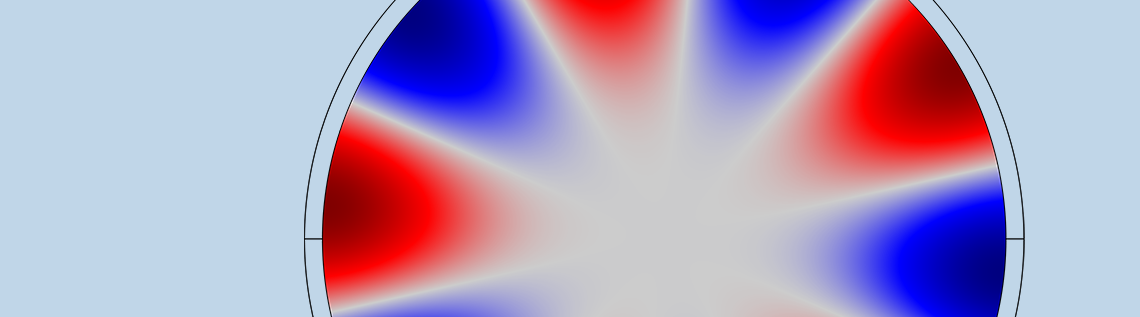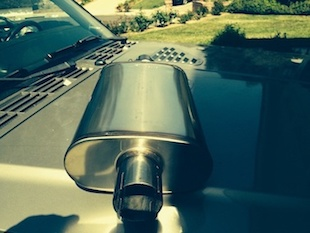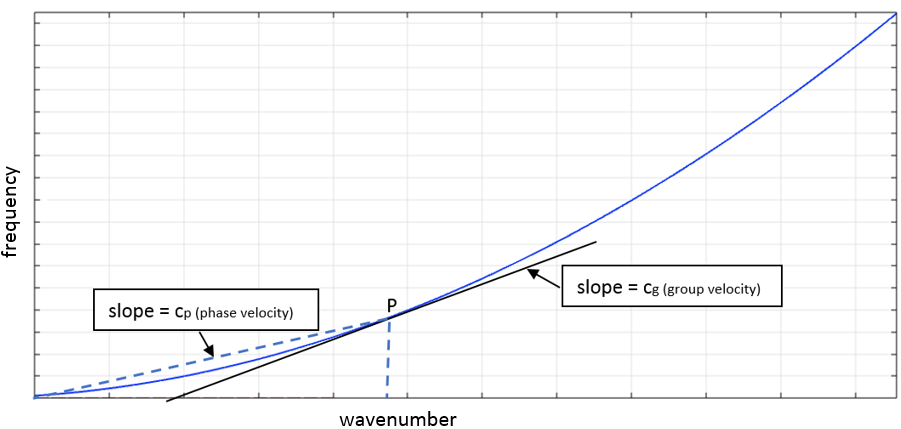# 利用色散曲线分析充液管道

2017年 11月 8日

### 充液管道：一个建模难题1. 特征模态
2. 波形式（或波模式）

### 利用色散曲线分析充液管道#### 空心管道与刚性壁管道的模态

(1)

\mu (z) \frac{\partial ^2 w}{\partial t^2}+L(z)w(z,t)=0

Eq. (1)中的 w(z,t)替换掉，可获得色散/特征方程。其解是成对出现的波数，表示波在±z 方向的运动。波数可表征为：

• 传播（k) 的纯实数值）
• 衰减（纯虚数 k
• 衰减振荡（k 的复值）

#### 结构模态

Timoshenko 等理论引入了剪切效应，可以准确地描述高频特性。对于复杂的横截面，解析解是不可行的。

#### 声学模式

p=\sum_{n=0}^{\infty}P_ncos(n\theta)J_n(k_rr)e^{(i\omega t-i k_zz)}

E = 2e11 N/m2
ρs = 7800 kg/m3
ν = 0.3
ρf = 1.25 kg/m3

ro = 0.05 m
t = 0.0025 m

### 计算充液管道的波模态

• 线弹性系统，或者在横截面方向而非长度方向上具有各向异性
• 恒定不变的横截面，适用于极长（无限长）波导
• 充分的有限元离散化，同时单元尺寸不宜太小，避免产生任何病态问题

### 参考文献

1. F. Fahy and P. Gardonio, Sound and Structural Vibration: Radiation, Transmission and Response, Elsevier Academic Press, 2007.
2. C.R. Fuller and F.J. Fahy, “Characteristics of wave propagation and energy distributions in cylindrical elastic shells filled with fluid,” Journal of Sound and Vibration, vol. 81, no. 501518, 1982.

3. A. Bhuddi, M.L. Gobert, and J.M. Mencik, “On the acoustic radiation of axisymmetric fluid-filled pipes using the Wave Finite Element (WFE) method,” Journal of Computational Acoustics, vol. 23, no. 3, 2015.

#### 评论 (2)

##### 留言##### HB Liao
2018-08-19##### Ajit Bhuddi
2018-08-20

To compute the forced response on a finite length waveguide, one can decompose the displacements in to the summation of wave modes, i.e. [q;F]= SUM_(n=1 to n) A1 e^{-ik_n*x}+A2 e^{i*k_n*x} ……….Eq(1), where A1 and A2 are the amplitudes of incident and reflected waves, respectively. k_n is the wave number for different types of wave. Based on the boundary conditions, A1 and A2 can be computed and the complete response can be computed using Eq(1).